Question

The shape of the distribution of the time required to get an oil change at a 20-minute oil-change facility is unknown. However, records indicate that the mean time is 21.4 minutes, and the standard deviation is 4.1 minutes. Complete parts (a) through (c).

(a) To compute probabilities regarding the sample mean using the normal model, what size sample would be required?

A. Any sample size could be used.

B. The sample size needs to be less than or equal to 30 .

C. The sample size needs to be greater than or equal to 30 .

D. The normal model cannot be used if the shape of the distribution is unknown.

(b) What is the probability that a random sample of n=35 oil changes results in a sample mean time less than 20 minutes?

The probability is approximately

(c) Suppose the manager agrees to pay each employee a \$ 50 bonus if they meet a certain goal. On a typical Saturday, the oil-change facility will perform 35 oil changes between 10 A.M. and 12 P.M. Treating this as a random sample, there would be a 10 % chance of the mean oil-change time being at or below what value? This will be the goal established by the manager.

There is a 10 % chance of being at or below a mean oil-change time of _______  minutes.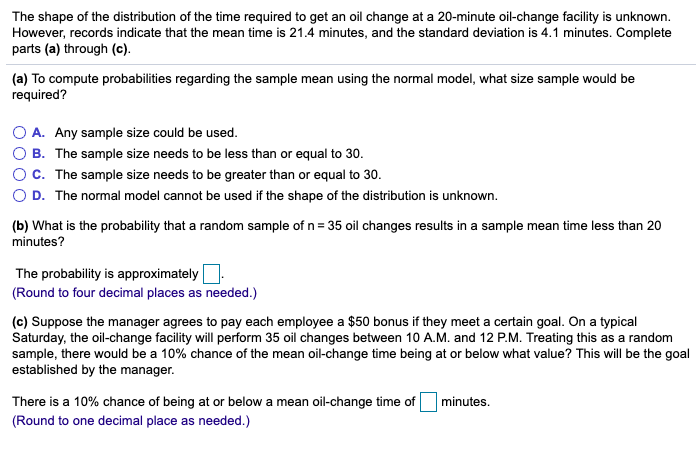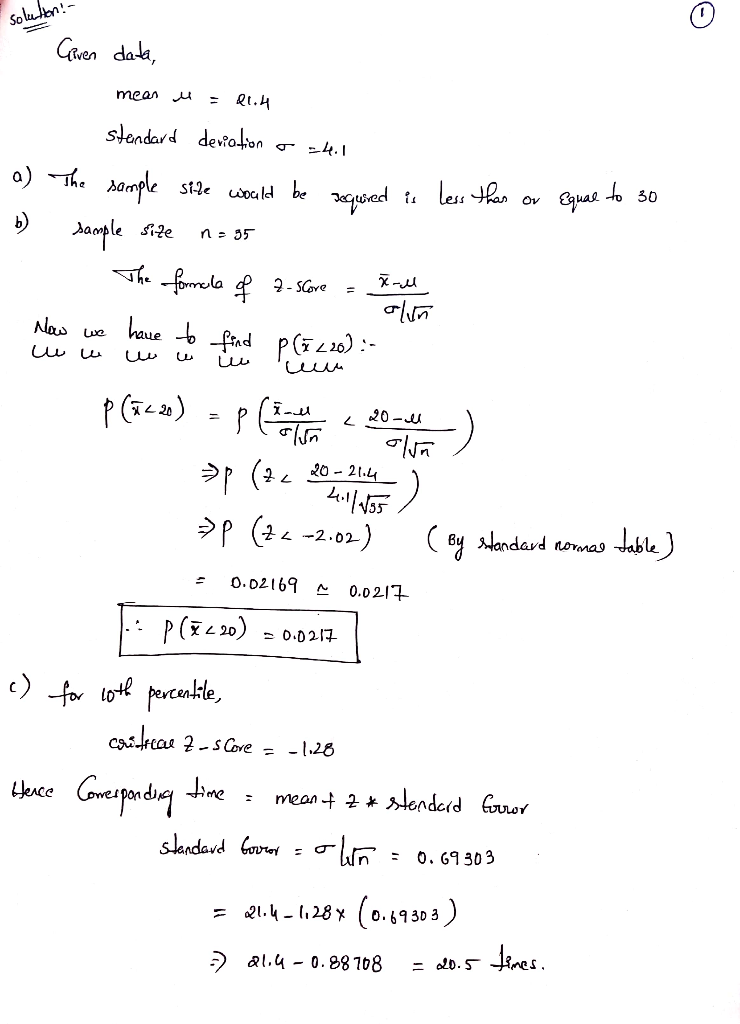#### Earn Coins

Coins can be redeemed for fabulous gifts.

Similar Homework Help Questions
• ### The shape of the distribution of the time required to get an oil change at a 10-minute oil-change facility is unknown....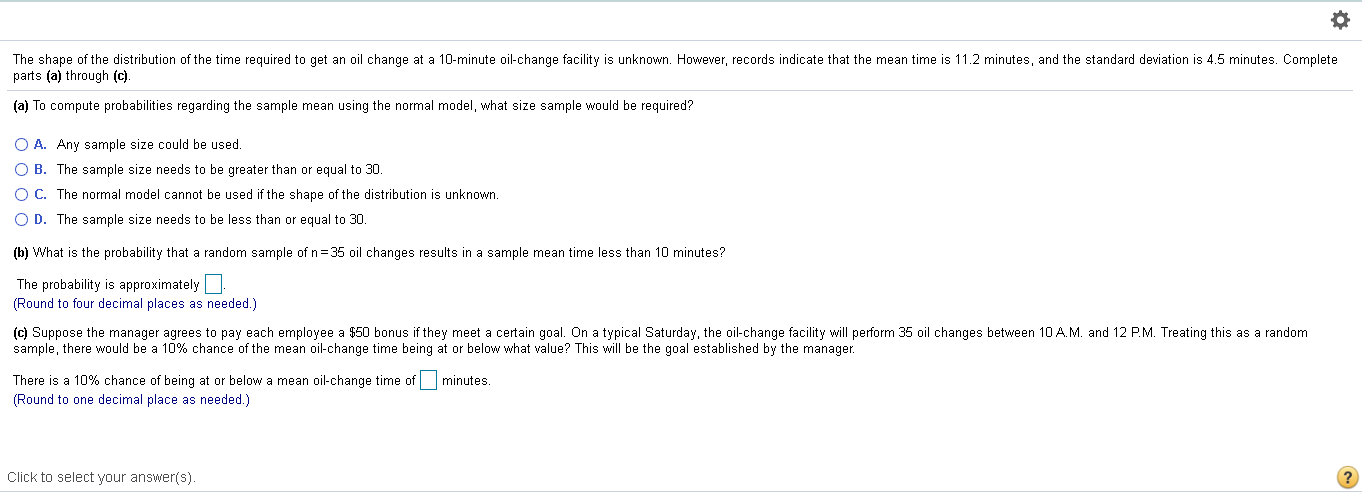The shape of the distribution of the time required to get an oil change at a 10-minute oil-change facility is unknown. However, records indicate that the mean time is 11.2 minutes, and the standard deviation is 4.5 minutes. Complete parts (a) through (c). (a) To compute probabilities regarding the sample mean using the normal model, what size sample would be required? A. Any sample size could be used. B. The sample size needs to be greater than or equal to 30. C. The normal...

• ### The shape of the distribution of the time required to get an oil change at a 20-minute oil change facility is unkno...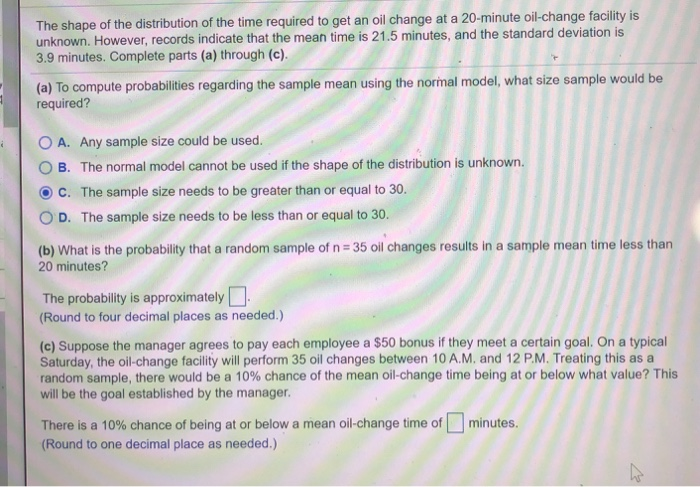The shape of the distribution of the time required to get an oil change at a 20-minute oil change facility is unknown. However, records indicate that the mean time is 21.5 minutes, and the standard deviation is 3.9 minutes. Complete parts (a) through (c). (a) To compute probabilities regarding the sample mean using the normal model, what size sample would be required? O A. Any sample size could be used. OB. The normal model cannot be used if the shape...

• ### The shape of the distribution of the time is required to get an oil change at a 15-minute oil-cha...

The shape of the distribution of the time is required to get an oil change at a 15-minute oil-change facility is unknown. However, records indicate that the mean time is 16.2 minutes, and the standard deviation is 4.2 minutes. Complete parts (a) through (c). (a) To compute probabilities regarding the sample mean using the normal model, what size sample would be required? A. The sample size needs to be less than or equal to 30. B. The sample size needs...

• ### The shape of the distribution of the time required to get an oil change at a...

The shape of the distribution of the time required to get an oil change at a 20-minute​ oil-change facility is unknown.​ However, records indicate that the mean time is 21.1 minutes and the standard deviation is 3.7 minutes ​(a) To compute probabilities regarding the sample mean using the normal​ model, what size sample would be​ required? A.Any sample size could be used. B.The sample size needs to be greater than or equal to 30. C.The sample size needs to be...

• ### The shape of the distribution of the time required to get an ail change at a 10-minute oil-change facility is skewed right.

The shape of the distribution of the time required to get an ail change at a 10-minute oil-change facility is skewed right. However, records indicate that the mean time is 11.3 minutes, and the standard deviation is 3.1 minutes. Complete parts (a) through (c). (a) To compute probabilities regarding the sample mean using the normal model, what size sample would be required? A. The normal model cannot be used if the shape of the distribution is skewed right. B. Any sample size could...

• ### The shape of the distribution of the time required to get an oil change at a...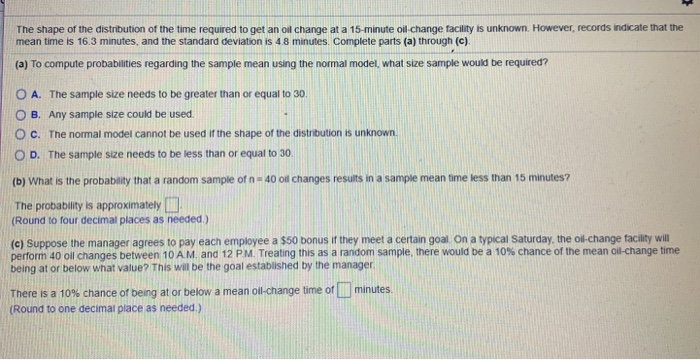The shape of the distribution of the time required to get an oil change at a 15-minute oil change facility is unknown. However, records indicate that the mean time is 16.3 minutes, and the standard deviation is 48 minutes. Complete parts (a) through (c) (a) To compute probabilities regarding the sample mean using the normal model, what size sample would be required? O A. The sample size needs to be greater than or equal to 30. O B. Any sample...

• ### The shape of the distribution of the time required to get an oil change at a...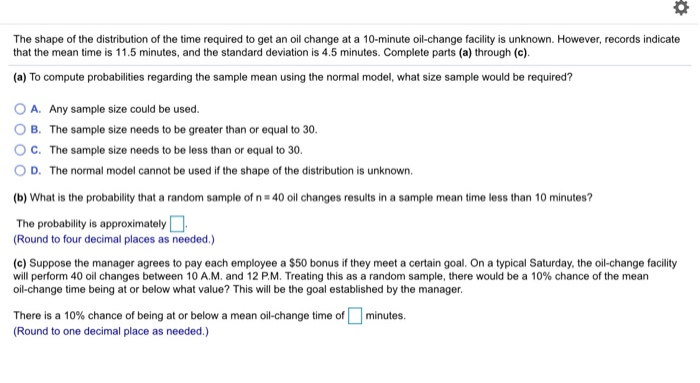The shape of the distribution of the time required to get an oil change at a 10-minute oil-change facility is unknown. However, records indicate that the mean time is 11.5 minutes, and the standard deviation is 4.5 minutes. Complete parts (a) through (c). (a) To compute probabilities regarding the sample mean using the normal model, what size sample would be required? O A. Any sample size could be used. B. The sample size needs to be greater than or equal...

• ### The shape of the distribution of the time required to get an oil change at a...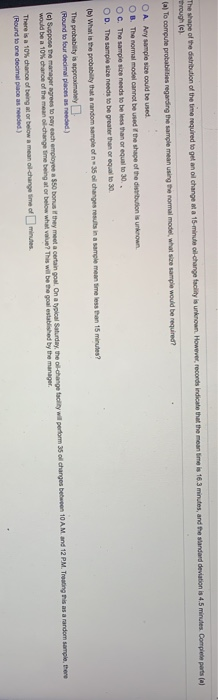The shape of the distribution of the time required to get an oil change at a 15-minute of change facility is unknown. However, records indicate that the mean time is 16.3 minutes, and the standard deviation is 4.5 minutes Complete parts al through (e) (a) To compute probabilities regarding the sample mean using the normal model, what size sample would be required? O A Any sample se could be used OB. The normal model cannot be used the shape of...

• ### The shape of the distribution of the time required to get an oil change at a...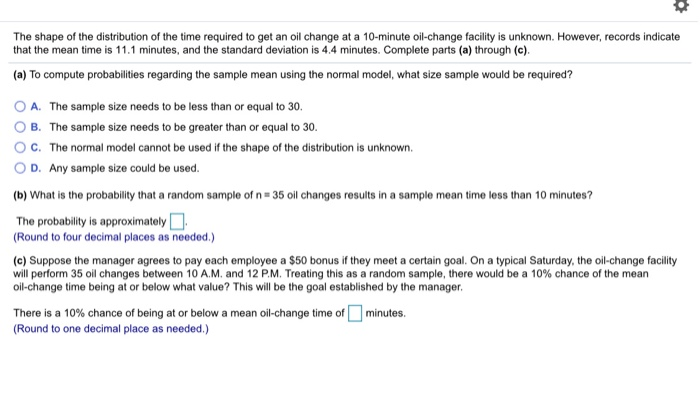The shape of the distribution of the time required to get an oil change at a 10-minute oil change facility is unknown. However, records indicate that the mean time is 11.1 minutes, and the standard deviation is 4.4 minutes. Complete parts (a) through (c). (a) To compute probabilities regarding the sample mean using the normal model, what size sample would be required? A. The sample size needs to be less than or equal to 30. B. The sample size needs...

• ### The shape of the distribution of the time required to get an oil change at a...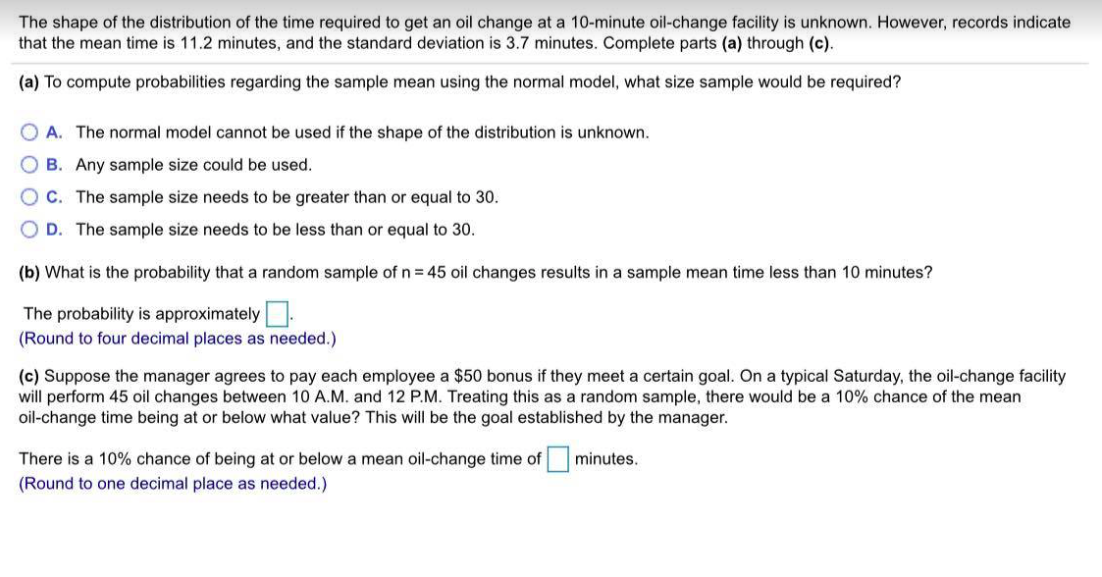The shape of the distribution of the time required to get an oil change at a 10-minute oil-change facility is unknown. However, records indicate that the mean time is 11.2 minutes, and the standard deviation is 3.7 minutes. Complete parts (a) through (c). (a) To compute probabilities regarding the sample mean using the normal model, what size sample would be required? O A. The normal model cannot be used if the shape of the distribution is unknown. OB. Any sample...